### Afternoondragon 02 Step 2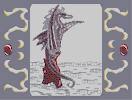Hover over the thumbnail for a full-size version.

Author Dragon_Moon author:dragon_moon incomplete rated 2006-11-26 4 by 24 people. \$Afternoondragon 02 Step 2#Dragon_Moon#none#1<6111196190619611119;111?1119;<18071;<6111@1119C11E?111111111@B11D611?111DN11:=1:=11PC111@11>9611>118718711A1196A118;111EN11;<1;<11PB111A118B11D>111111111AC11E7111>1118:=19061:=7111A111=7111187180718711118:1|12^254,502!12^257,494!12^259,486!12^262,478!12^263,471!12^265,462!12^265,450!12^264,438!12^262,425!12^260,413!12^267,411!12^271,405!12^269,395!12^269,384!12^267,374!12^267,364!12^267,357!12^275,359!12^373,522!12^370,514!12^369,505!12^363,478!12^359,431!12^356,417!12^349,410!12^335,382!12^335,369!12^342,341!12^348,334!12^354,327!12^346,317!12^333,320!12^352,323!12^337,355!12^337,366!12^255,499!12^255,499!12^255,496!12^256,493!12^257,491!12^258,489!12^260,485!12^260,485!12^261,482!12^262,478!12^263,476!12^264,474!12^264,472!12^265,467!12^265,466!12^266,463!12^266,460!12^266,457!12^266,453!12^266,452!12^266,448!12^266,444!12^265,441!12^264,437!12^263,433!12^263,429!12^262,425!12^261,423!12^261,421!12^260,418!12^259,416!12^259,414!12^259,414!12^260,412!12^262,410!12^265,407!12^268,405!12^271,402!12^271,398!12^271,393!12^271,389!12^271,385!12^270,381!12^270,377!12^270,373!12^269,370!12^269,364!12^269,361!12^270,360!12^272,360!12^274,360!12^275,360!12^374,522!12^374,520!12^372,513!12^371,512!12^370,507!12^370,502!12^367,496!12^365,493!12^365,488!12^362,479!12^361,475!12^361,471!12^361,465!12^361,464!12^360,437!12^358,432!12^358,429!12^358,423!12^357,419!12^354,414!12^351,409!12^349,405!12^345,398!12^344,397!12^342,394!12^341,391!12^340,387!12^339,383!12^339,378!12^338,374!12^338,373!12^338,370!12^341,346!12^341,341!12^344,338!12^348,334!12^353,329!12^356,326!12^354,322!12^352,319!12^346,316!12^343,318!12^338,320!12^334,320!12^330,321!12^333,363!12^336,357!12^338,352!12^266,355!12^265,354!12^264,353!12^264,353!12^298,314!12^298,313!12^294,297!12^287,270!12^286,239!12^287,204!12^292,155!12^317,85!12^349,34!12^297,310!12^297,305!12^295,299!12^292,292!12^288,283!12^287,279!12^287,273!12^287,265!12^287,260!12^287,253!12^287,246!12^287,239!12^287,234!12^287,230!12^288,221!12^288,214!12^288,208!12^289,202!12^289,196!12^289,189!12^289,186!12^290,182!12^291,179!12^292,175!12^292,172!12^292,167!12^292,161!12^293,157!12^294,155!12^294,152!12^295,148!12^296,139!12^297,134!12^297,126!12^299,121!12^300,117!12^307,105!12^308,101!12^308,95!12^312,90!12^313,88!12^315,83!12^321,76!12^324,70!12^327,64!12^351,33!12^297,313!12^297,312!12^297,309!12^296,307!12^296,306!12^295,304!12^293,302!12^292,300!12^291,299!12^290,296!12^290,294!12^289,292!12^289,289!12^287,286!12^286,285!12^286,282!12^286,279!12^286,273!12^286,269!12^286,266!12^286,260!12^286,255!12^286,249!12^286,246!12^286,242!12^286,237!12^286,232!12^286,230!12^286,227!12^286,225!12^287,220!12^287,219!12^287,216!12^287,211!12^287,210!12^287,205!12^287,202!12^288,200!12^288,199!12^288,196!12^288,192!12^288,190!12^288,186!12^288,183!12^288,180!12^290,179!12^290,177!12^290,176!12^290,175!12^290,172!12^291,171!12^291,169!12^291,168!12^291,166!12^291,163!12^291,162!12^293,159!12^293,157!12^293,154!12^294,151!12^294,151!12^295,149!12^295,148!12^295,147!12^295,145!12^295,142!12^295,139!12^296,135!12^296,132!12^296,131!12^296,129!12^297,128!12^298,126!12^298,124!12^298,122!12^300,120!12^305,109!12^305,108!12^305,104!12^302,115!12^302,113!12^302,112!12^302,112!12^302,110!12^302,109!12^306,101!12^307,98!12^312,87!12^315,85!12^316,83!12^318,80!12^319,77!12^320,74!12^322,72!12^323,70!12^325,67!12^326,65!12^329,62!12^330,60!12^331,58!12^334,55!12^335,51!12^337,49!12^337,47!12^339,44!12^341,43!12^343,41!12^346,38!12^347,36!12^348,35!12^334,54!12^334,54!12^309,94!12^312,91!12^311,94!12^311,91!12^313,88!12^329,104!12^325,134!12^327,161!12^332,188!12^337,213!12^346,243!12^340,296!12^327,289!12^321,298!12^307,296!12^362,279!12^347,278!12^348,39!12^344,44!12^343,49!12^341,52!12^340,56!12^339,59!12^337,63!12^337,67!12^337,71!12^334,79!12^333,83!12^333,86!12^331,92!12^331,94!12^331,97!12^330,100!12^326,117!12^326,123!12^327,126!12^327,128!12^327,131!12^327,134!12^327,138!12^328,142!12^329,146!12^329,149!12^329,153!12^329,157!12^329,160!12^328,108!12^328,110!12^328,112!12^328,114!12^329,165!12^329,168!12^329,172!12^331,175!12^331,177!12^331,180!12^332,184!12^332,187!12^334,189!12^334,192!12^334,194!12^334,195!12^336,197!12^336,200!12^337,203!12^337,206!12^338,209!12^338,215!12^339,217!12^341,220!12^343,222!12^343,226!12^344,228!12^344,230!12^345,233!12^345,235!12^346,237!12^347,238!12^347,241!12^347,243!12^348,248!12^350,249!12^352,251!12^353,253!12^355,257!12^357,259!12^357,262!12^358,265!12^359,265!12^360,268!12^361,271!12^361,273!12^362,275!12^363,277!12^340,214!12^340,214!12^327,119!12^359,277!12^357,276!12^353,276!12^351,277!12^347,280!12^345,284!12^344,286!12^342,289!12^342,294!12^337,288!12^336,288!12^333,287!12^328,288!12^325,289!12^323,291!12^323,294!12^323,297!12^321,298!12^320,298!12^316,296!12^313,295!12^309,295!12^307,296!12^304,301!12^302,304!12^302,308!12^334,73!12^336,73!12^333,79!12^330,83!12^330,88!12^330,92!12^330,95!12^353,28!12^351,30!12^350,34!12^348,36!12^326,304!12^329,300!12^331,296!12^330,300!12^324,305!12^333,292!12^357,286!12^356,292!12^352,302!12^352,305!12^351,309!12^357,310!12^363,311!12^363,314!12^363,318!12^348,310!12^342,310!12^340,310!12^337,311!12^333,315!12^331,316!12^340,306!12^343,301!12^343,299!12^355,297!12^353,301!12^358,283!12^362,257!12^362,253!12^362,249!12^360,245!12^357,240!12^354,236!12^352,233!12^350,232!12^348,232!12^362,262!12^363,239!12^368,232!12^369,229!12^369,225!12^369,220!12^366,216!12^363,216!12^360,216!12^355,219!12^354,223!12^354,226!12^354,230!12^367,235!12^366,209!12^367,203!12^369,198!12^370,193!12^370,187!12^370,183!12^369,177!12^366,175!12^363,174!12^359,176!12^357,177!12^354,180!12^352,182!12^351,185!12^351,188!12^351,189!12^351,193!12^351,191!12^351,196!12^351,200!12^353,205!12^355,207!12^355,209!12^357,212!12^358,212!12^374,213!12^374,210!12^376,206!12^377,203!12^380,197!12^382,192!12^383,189!12^383,185!12^383,182!12^382,179!12^379,176!12^376,174!12^372,173!12^368,176!12^373,213!12^374,213!12^371,216!12^377,168!12^378,162!12^378,156!12^376,149!12^374,146!12^373,143!12^371,139!12^368,137!12^366,134!12^363,131!12^359,126!12^357,123!12^356,119!12^356,113!12^356,108!12^358,104!12^358,103!12^361,101!12^366,100!12^370,100!12^374,100!12^381,103!12^387,107!12^390,107!12^395,107!12^399,107!12^404,107!12^411,107!12^412,107!12^414,107!12^387,108!12^386,107!12^381,104!12^380,104!12^376,101!12^408,108!12^419,106!12^424,106!12^428,105!12^431,104!12^434,102!12^435,99!12^432,97!12^429,95!12^426,93!12^421,93!12^419,93!12^416,93!12^413,93!12^409,93!12^404,92!12^402,90!12^397,87!12^393,84!12^388,81!12^383,79!12^380,78!12^374,78!12^369,78!12^367,78!12^364,78!12^360,78!12^355,79!12^351,82!12^347,84!12^344,86!12^341,88!12^338,93!12^337,97!12^335,102!12^335,107!12^335,111!12^335,114!12^336,119!12^337,121!12^338,124!12^339,126!12^342,130!12^342,133!12^342,138!12^334,189!12^388,88!12^385,86!12^382,85!12^380,85!12^376,85!12^374,85!12^373,85!12^360,78!12^345,85!12^334,177!12^262,193!12^257,196!12^251,199!12^246,203!12^241,207!12^235,210!12^231,214!12^226,218!12^221,221!12^214,227!12^209,231!12^219,224!12^221,268!12^226,263!12^229,260!12^234,255!12^240,251!12^245,247!12^252,242!12^258,237!12^263,232!12^270,227!12^276,223!12^282,220!12^250,300!12^252,294!12^258,287!12^263,280!12^269,274!12^273,268!12^278,261!12^402,142!12^382,149!12^389,146!12^397,143!12^341,142!12^342,145!12^341,149!12^341,152!12^341,154!12^340,157!12^338,158!12^337,161!12^335,162!12^334,164!12^333,167!12^333,168!12^267,190!12^274,187!12^410,140!12^418,137!12^427,134!12^437,132!12^446,130!12^455,129!12^463,128!12^472,128!12^386,189!12^395,183!12^404,175!12^413,170!12^422,163!12^430,157!12^439,152!12^446,146!12^454,142!12^462,137!12^470,134!12^479,129!12^488,129!12^499,131!12^480,132!12^489,132!12^385,164!12^390,186!12^400,180!12^412,171!12^419,165!12^318,303!12^322,311!12^329,319!12^332,321!12^335,326!12^335,329!12^336,332!12^336,335!12^328,343!12^326,343!12^322,343!12^319,343!12^316,342!12^313,339!12^311,337!12^309,335!12^307,332!12^302,326!12^302,323!12^301,320!12^300,318!12^305,341!12^305,347!12^307,351!12^309,356!12^313,358!12^315,363!12^316,369!12^316,373!12^317,375!12^325,350!12^326,353!12^327,357!12^327,360!12^327,364!12^327,366!12^327,371!12^310,338!12^327,318!12^323,313!12^318,307!2^416,503,-0.707106781186547,-0.707106781186547!2^412,509,-1,0!2^413,505,-0.707106781186547,-0.707106781186547!2^415,516,-0.707106781186547,0.707106781186547!2^423,520,0,1!2^437,522,-0.707106781186547,-0.707106781186547!2^435,518,-0.707106781186547,-0.707106781186547!2^444,513,-0.707106781186547,-0.707106781186547!2^442,507,-1,0!2^444,501,1,0!2^442,490,0.707106781186547,-0.707106781186547!2^436,500,1,0!2^432,489,0.707106781186547,-0.707106781186547!2^432,481,0.707106781186547,-0.707106781186547!2^421,483,0,-1!2^420,477,0,-1!2^415,474,0,-1!2^403,472,0.707106781186547,-0.707106781186547!2^408,480,0,-1!2^395,475,0.707106781186547,-0.707106781186547!2^393,472,0,-1!2^393,468,0,-1!2^392,463,0.707106781186547,-0.707106781186547!2^407,471,0,-1!2^392,471,0,1!2^380,472,0.707106781186547,-0.707106781186547!2^383,458,0,-1!2^374,455,0.707106781186547,-0.707106781186547!2^376,456,0,-1!2^372,467,0,-1!2^375,471,0,-1!2^360,462,0,-1!2^348,456,0.707106781186547,-0.707106781186547!2^364,464,0.707106781186547,-0.707106781186547!2^365,451,0,-1!2^355,443,0.707106781186547,-0.707106781186547!2^360,448,0,-1!2^347,432,0.707106781186547,-0.707106781186547!2^349,438,0.707106781186547,-0.707106781186547!2^338,449,0.707106781186547,-0.707106781186547!2^330,442,0.707106781186547,-0.707106781186547!2^322,432,0.707106781186547,-0.707106781186547!2^313,424,0.707106781186547,-0.707106781186547!2^340,428,0.707106781186547,-0.707106781186547!2^335,420,0.707106781186547,-0.707106781186547!2^308,413,0.707106781186547,-0.707106781186547!2^307,414,0.707106781186547,-0.707106781186547!2^314,420,0.707106781186547,-0.707106781186547!2^299,404,0.707106781186547,-0.707106781186547!2^303,415,0.707106781186547,-0.707106781186547!2^301,404,-1,0!2^296,396,-1,0!2^292,386,-1,0!2^293,394,-0.707106781186547,0.707106781186547!2^298,402,-0.707106781186547,0.707106781186547!2^284,383,1,0!2^282,375,1,0!2^280,363,1,0!2^279,355,1,0!2^282,379,1,0!2^280,367,1,0!2^279,358,1,0!2^280,346,0.707106781186547,-0.707106781186547!2^274,337,1,0!2^278,348,1,0!2^276,343,1,0!2^273,329,1,0!2^272,315,1,0!2^271,308,1,0!2^330,412,0.707106781186547,-0.707106781186547!2^323,405,0.707106781186547,-0.707106781186547!2^358,444,0.707106781186547,-0.707106781186547!2^402,466,0,-1!2^411,469,0.707106781186547,-0.707106781186547!2^392,464,0,-1!2^315,395,0.707106781186547,-0.707106781186547!2^306,384,0.707106781186547,-0.707106781186547!2^301,376,1,0!2^305,385,1,0!2^299,366,1,0!2^298,356,1,0!2^296,346,1,0!2^299,333,1,0!2^276,297,-1,0!2^278,283,-0.707106781186547,-0.707106781186547!2^275,288,-1,0!2^280,280,-1,0!2^282,279,-1,0!2^278,282,-0.707106781186547,-0.707106781186547!2^443,502,1,0!2^439,499,1,0!2^437,492,0.707106781186547,-0.707106781186547!2^402,475,0,-1!2^380,466,0,-1!2^374,464,0,-1!2^369,459,0,-1!2^353,455,0.707106781186547,-0.707106781186547!2^360,452,0.707106781186547,-0.707106781186547!2^372,461,0.707106781186547,-0.707106781186547!2^343,446,0.707106781186547,-0.707106781186547!2^350,444,0.707106781186547,-0.707106781186547!2^335,437,0.707106781186547,-0.707106781186547!2^340,433,0.707106781186547,-0.707106781186547!2^323,428,0.707106781186547,-0.707106781186547!2^328,425,0.707106781186547,-0.707106781186547!2^331,423,0.707106781186547,-0.707106781186547!2^316,418,0.707106781186547,-0.707106781186547!2^321,416,0.707106781186547,-0.707106781186547!2^323,411,0.707106781186547,-0.707106781186547!2^307,411,0.707106781186547,-0.707106781186547!2^309,408,0.707106781186547,-0.707106781186547!2^312,404,0.707106781186547,-0.707106781186547!2^309,395,0.707106781186547,-0.707106781186547!2^298,398,0.707106781186547,-0.707106781186547!2^300,393,0.707106781186547,-0.707106781186547!2^290,389,0.707106781186547,-0.707106781186547!2^289,384,0.707106781186547,-0.707106781186547!2^293,381,0.707106781186547,-0.707106781186547!2^298,378,0.707106781186547,-0.707106781186547!2^302,386,0.707106781186547,-0.707106781186547!2^286,370,1,0!2^294,366,1,0!2^291,364,1,0!2^284,356,1,0!2^292,352,1,0!2^290,352,0.707106781186547,-0.707106781186547!2^290,344,0.707106781186547,-0.707106781186547!2^284,342,0.707106781186547,-0.707106781186547!2^293,335,0.707106781186547,-0.707106781186547!2^295,329,0.707106781186547,-0.707106781186547!2^283,334,0.707106781186547,-0.707106781186547!2^282,324,0.707106781186547,-0.707106781186547!2^286,317,0.707106781186547,-0.707106781186547!2^285,308,0.707106781186547,-0.707106781186547!2^278,311,0.707106781186547,-0.707106781186547!2^277,300,0.707106781186547,-0.707106781186547!2^277,292,1,0!2^283,297,1,0!2^294,306,1,0!2^289,297,1,0!2^286,289,1,0!2^284,280,1,0!2^284,268,1,0!2^284,256,1,0!2^284,244,1,0!2^284,233,1,0!2^284,223,1,0!2^284,210,1,0!2^284,197,1,0!2^285,190,1,0!2^286,179,1,0!2^287,171,1,0!2^290,162,1,0!2^292,154,1,0!2^292,147,1,0!2^294,136,1,0!2^297,127,1,0!2^298,117,1,0!2^303,288,-1,0!2^302,278,-1,0!2^299,266,-1,0!2^299,257,-1,0!2^299,248,-1,0!2^300,236,-1,0!2^307,286,1,0!2^302,286,1,0!2^303,278,1,0!2^300,272,1,0!2^300,257,1,0!2^300,248,1,0!2^297,236,1,0!2^295,228,1,0!2^294,217,1,0!2^295,203,1,0!2^295,194,1,0!2^296,186,1,0!2^296,173,1,0!2^295,160,1,0!2^298,148,1,0!10^277,283!10^276,289!10^276,296!10^276,302!10^277,310!10^277,316!10^277,324!10^279,330!10^280,337!10^282,345!10^282,350!10^284,358!10^287,365!10^285,370!10^287,376!10^286,331!10^286,324!10^283,318!10^283,306!10^287,301!10^283,294!10^288,315!10^290,323!10^294,329!10^288,337!10^288,346!10^288,354!10^290,362!10^291,371!10^294,378!10^297,384!10^303,391!10^307,396!10^297,392!10^290,382!10^288,390!10^295,398!10^302,404!10^306,410!10^314,416!10^309,405!10^302,401!10^318,422!10^325,427!10^323,418!10^317,410!10^343,449!10^337,443!10^334,437!10^329,430!10^339,436!10^334,428!10^344,441!10^349,445!10^350,450!10^355,451!10^359,453!10^367,455!10^372,457!10^377,459!10^383,462!10^391,466!10^398,468!10^411,473!10^405,472!10^418,474!3^358,444!3^349,437!3^342,429!3^332,417!3^324,408!3^310,390!3^302,378!3^299,360!3^298,347!3^298,335!3^283,377!3^283,368!3^282,357!3^279,346!3^278,338!3^279,277!3^360,459!3^379,467!3^402,475!3^391,460!3^426,480!3^434,482!3^442,489!3^443,500!3^440,513!3^429,521!3^406,511!10^307,289!10^304,283!10^303,272!10^300,263!10^300,252!10^300,241!10^298,230!10^298,222!10^299,207!3^300,196!3^298,187!3^298,171!3^299,158!3^300,146!10^298,215!10^302,279!10^301,257!2^301,315,-0.707106781186547,0.707106781186547!2^304,309,-1,0!2^308,302,-0.707106781186547,-0.707106781186547!2^314,301,0.707106781186547,-0.707106781186547!2^322,301,-1,0!2^327,293,-0.707106781186547,-0.707106781186547!2^336,291,0.707106781186547,-0.707106781186547!2^340,298,1,0!2^347,288,-0.707106781186547,-0.707106781186547!2^352,281,-0.707106781186547,-0.707106781186547!2^358,281,0,-1!2^362,273,-1,0!2^359,258,-0.707106781186547,0.707106781186547!2^350,248,-1,0!2^353,250,-0.707106781186547,0.707106781186547!2^348,238,-1,0!2^347,228,-0.707106781186547,0.707106781186547!2^342,221,-1,0!2^341,211,-1,0!2^339,202,-1,0!2^335,191,-1,0!2^333,182,-1,0!2^331,172,-1,0!2^330,165,-1,0!2^332,155,-1,0!2^331,146,-1,0!2^329,134,-1,0!2^329,121,-1,0!2^331,111,-1,0!2^332,102,-1,0!2^333,92,-1,0!2^335,81,-1,0!2^340,62,-1,0!2^342,54,-0.707106781186547,-0.707106781186547!2^347,44,-1,0!2^349,34,-0.707106781186547,-0.707106781186547!2^348,41,-0.707106781186547,-0.707106781186547!2^306,108,-1,0!2^309,101,-0.707106781186547,-0.707106781186547!2^311,95,-1,0!2^314,88,-0.707106781186547,-0.707106781186547!2^319,81,-0.707106781186547,-0.707106781186547!2^322,72,-0.707106781186547,-0.707106781186547!2^330,61,-0.707106781186547,-0.707106781186547!2^336,52,-0.707106781186547,-0.707106781186547!2^342,43,-0.707106781186547,-0.707106781186547!2^299,138,1,0!2^304,128,1,0!2^304,115,1,0!2^305,105,1,0!2^314,94,-1,0!2^316,85,-1,0!2^319,79,-1,0!10^303,294!10^324,283!10^321,274!10^320,264!10^318,256!10^317,246!10^315,240!10^313,231!10^314,224!10^314,215!10^312,207!10^312,201!10^312,194!10^311,186!10^310,181!10^310,174!10^309,167!10^309,160!10^309,155!10^309,150!10^309,143!10^308,137!10^323,279!10^320,269!10^319,261!10^318,252!10^318,244!10^314,234!10^313,226!10^314,219!10^312,212!10^313,202!10^312,197!10^312,190!10^311,178!10^310,172!10^309,164!10^324,290!10^332,283!10^330,276!10^328,268!10^326,259!10^324,250!10^321,241!10^319,233!10^318,224!10^318,216!10^317,207!10^303,136!10^306,127!10^309,117!10^310,109!10^313,102!10^317,92!10^319,84!10^323,75!10^328,67!10^332,60!3^330,278!3^325,270!3^324,261!3^323,254!3^321,246!3^347,275!3^345,267!3^342,260!3^341,250!3^338,241!3^336,232!3^334,220!3^333,209!3^330,197!3^329,187!3^327,179!3^327,170!3^327,159!3^325,148!3^325,137!3^323,128!3^322,117!3^323,103!3^328,87!10^315,135!10^315,127!10^315,115!10^316,102!10^320,93!10^315,144!10^312,122!10^312,152!10^315,107!3^349,273!3^348,266!3^346,258!3^344,249!3^342,237!3^339,224!3^339,213!3^336,197!3^334,184!3^331,166!3^329,152!3^323,111!3^336,52!3^339,43!3^346,38!2^289,296,0.707106781186547,-0.707106781186547!12^48,336!12^42,336!12^36,336!12^36,330!12^30,330!12^30,324!12^24,324!12^24,312!12^24,318!12^24,306!12^30,306!12^36,312!12^42,312!12^42,318!12^48,318!12^54,324!12^54,330!12^54,336!12^750,336!12^750,330!12^750,324!12^744,318!12^738,318!12^738,312!12^732,312!12^726,306!12^720,306!12^720,312!12^720,318!12^720,324!12^726,324!12^726,330!12^732,330!12^732,336!12^738,336!12^744,336!12^48,264!12^54,264!12^60,270!12^60,264!12^66,270!12^66,276!12^72,276!12^72,282!12^72,288!12^72,294!12^72,300!12^72,306!12^72,312!12^66,312!12^60,306!12^60,300!12^60,294!12^54,288!12^48,282!12^42,282!12^36,282!12^30,282!12^24,282!12^24,276!12^30,270!12^36,264!12^42,264!12^768,312!12^762,312!12^756,306!12^756,300!12^756,294!12^768,306!12^768,300!12^768,294!12^768,288!12^768,282!12^750,288!12^744,282!12^732,282!12^738,282!12^726,282!12^720,282!12^720,276!12^726,270!12^732,264!12^738,264!12^744,264!12^750,264!12^756,264!12^756,270!12^762,270!12^762,276!12^768,276!12^24,300!12^24,294!12^24,288!12^30,288!12^36,288!12^42,288!12^48,288!12^54,294!12^54,306!12^54,300!12^48,300!12^48,294!12^42,294!12^36,294!12^30,294!12^30,300!12^36,300!12^42,300!12^36,306!12^42,306!12^48,306!12^48,312!12^54,312!12^60,312!12^60,318!12^54,318!12^66,318!12^72,318!12^66,324!12^60,324!12^60,330!12^66,330!12^60,336!12^720,300!12^720,294!12^720,288!12^732,288!12^726,288!12^726,294!12^732,294!12^744,294!12^738,294!12^738,288!12^744,288!12^750,294!12^750,300!12^744,300!12^738,300!12^732,300!12^726,300!12^732,306!12^738,306!12^744,306!12^750,306!12^750,312!12^744,312!12^756,312!12^756,318!12^756,324!12^750,318!12^762,318!12^768,318!12^762,324!12^756,330!12^762,330!12^756,336!12^27,273!12^33,267!12^38,266!12^55,267!12^49,265!12^63,273!12^68,281!12^69,288!12^70,293!12^63,308!12^62,304!12^61,300!12^58,290!12^52,284!12^45,280!12^40,280!12^36,281!12^41,332!12^33,327!12^26,320!12^32,310!12^38,315!12^46,320!12^51,323!12^723,272!12^730,268!12^735,266!12^752,268!12^747,266!12^758,274!12^764,281!12^765,286!12^766,292!12^760,308!12^758,305!12^758,302!12^757,298!12^754,291!12^747,283!12^742,280!12^737,280!12^733,282!12^762,280!12^724,320!12^730,327!12^736,333!12^739,335!12^746,332!12^745,321!12^741,320!12^734,315!12^729,309!3^38,334!3^29,327!3^22,319!3^46,337!3^56,334!3^63,328!3^68,320!3^71,316!3^56,323!3^46,317!3^40,313!3^31,308!3^23,309!3^26,297!3^59,313!3^47,308!3^35,299!3^25,286!3^60,324!3^62,328!3^45,336!3^34,332!3^24,322!3^21,306!3^26,299!3^760,326!3^756,332!3^748,335!3^738,337!3^732,334!3^725,329!3^722,324!3^719,317!3^719,311!3^720,305!3^722,294!3^729,300!3^732,308!3^741,315!3^749,317!3^743,303!3^739,305!3^729,292!3^753,309!3^758,318!3^766,317!3^754,322!3^753,333!3^735,335!3^725,327!3^718,314!3^741,312!3^57,333!2^212,233,-0.707106781186547,-0.707106781186547!2^221,226,-0.707106781186547,-0.707106781186547!2^232,218,-0.707106781186547,-0.707106781186547!2^241,208,-0.707106781186547,-0.707106781186547!2^252,203,0,-1!2^257,199,-0.707106781186547,-0.707106781186547!2^266,194,0,-1!2^278,188,-0.707106781186547,-0.707106781186547!2^285,184,0,-1!2^337,72,-0.707106781186547,-0.707106781186547!2^225,269,-0.707106781186547,-0.707106781186547!2^235,262,-0.707106781186547,-0.707106781186547!2^242,254,-0.707106781186547,-0.707106781186547!2^251,246,-0.707106781186547,-0.707106781186547!2^262,237,-0.707106781186547,-0.707106781186547!2^272,230,-0.707106781186547,-0.707106781186547!2^282,223,-0.707106781186547,-0.707106781186547!2^254,299,-0.707106781186547,-0.707106781186547!2^262,288,-0.707106781186547,-0.707106781186547!2^269,277,-0.707106781186547,-0.707106781186547!2^277,268,-0.707106781186547,-0.707106781186547!2^283,259,-0.707106781186547,-0.707106781186547!2^257,287,-0.707106781186547,-0.707106781186547!2^261,277,-1,0!2^258,265,-0.707106781186547,0.707106781186547!2^247,260,0,1!2^233,261,0.707106781186547,0.707106781186547!2^234,254,-0.707106781186547,-0.707106781186547!2^240,244,-1,0!2^235,233,-0.707106781186547,0.707106781186547!2^223,227,0,1!2^204,240,-0.707106781186547,-0.707106781186547!2^266,273,-0.707106781186547,-0.707106781186547!2^264,266,-0.707106781186547,-0.707106781186547!2^260,258,-0.707106781186547,-0.707106781186547!2^254,253,-0.707106781186547,-0.707106781186547!2^244,256,-0.707106781186547,-0.707106781186547!2^254,257,-0.707106781186547,-0.707106781186547!2^247,243,-0.707106781186547,-0.707106781186547!2^247,236,-0.707106781186547,-0.707106781186547!2^245,231,-0.707106781186547,-0.707106781186547!2^237,228,-0.707106781186547,-0.707106781186547!2^229,223,-0.707106781186547,-0.707106781186547!2^233,224,-0.707106781186547,-0.707106781186547!2^237,225,-0.707106781186547,-0.707106781186547!2^257,227,-0.707106781186547,-0.707106781186547!2^253,224,-0.707106781186547,-0.707106781186547!2^243,224,-0.707106781186547,-0.707106781186547!2^271,261,-0.707106781186547,-0.707106781186547!2^266,254,-0.707106781186547,-0.707106781186547!2^266,259,-0.707106781186547,-0.707106781186547!10^224,223!10^229,217!10^236,213!10^242,208!10^247,204!10^253,201!10^262,198!10^269,194!10^276,190!10^280,187!10^278,193!10^274,198!10^266,199!10^258,203!10^249,208!10^238,216!10^213,229!10^251,214!10^257,212!10^264,209!10^268,207!10^273,205!10^279,201!10^279,208!10^276,214!10^274,216!10^267,218!10^262,220!10^257,230!10^257,224!10^253,233!10^228,266!10^233,263!10^241,260!10^247,258!10^250,255!10^253,251!10^255,247!10^259,246!10^263,246!10^266,247!10^269,250!10^272,252!10^249,206!10^258,206!10^265,205!10^267,201!10^272,197!10^243,211!10^235,216!10^271,267!10^272,261!10^275,257!10^278,254!10^278,246!10^278,243!10^274,242!10^268,240!10^264,240!10^273,238!10^276,236!10^278,232!10^279,238!3^240,229!3^244,226!3^205,235!3^228,260!3^234,255!3^237,234!3^256,289!3^264,276!3^278,229!2^362,216,0,-1!2^356,212,0.707106781186547,-0.707106781186547!2^349,202,1,0!2^347,192,1,0!2^350,179,0.707106781186547,0.707106781186547!2^361,174,0,1!2^373,181,-1,0!2^372,195,-1,0!2^368,210,-0.707106781186547,-0.707106781186547!2^374,213,-0.707106781186547,-0.707106781186547!2^379,203,-0.707106781186547,-0.707106781186547!2^386,191,-1,0!2^384,180,-0.707106781186547,0.707106781186547!2^374,171,0,1!2^359,223,-0.707106781186547,-0.707106781186547!2^354,231,-1,0!2^361,240,-0.707106781186547,0.707106781186547!2^367,238,-0.707106781186547,-0.707106781186547!2^372,225,-1,0!2^381,165,-1,0!2^381,154,-1,0!2^376,144,-0.707106781186547,0.707106781186547!2^368,135,-0.707106781186547,0.707106781186547!2^360,126,-1,0!2^358,117,-1,0!2^359,108,-0.707106781186547,-0.707106781186547!2^367,102,0,-1!2^377,105,0.707106781186547,-0.707106781186547!2^388,111,0,-1!2^398,111,0,-1!2^410,111,0,-1!2^421,109,0,-1!2^433,108,-0.707106781186547,-0.707106781186547!2^434,96,-0.707106781186547,0.707106781186547!2^422,92,0,1!2^413,92,0,1!2^404,86,-0.707106781186547,0.707106781186547!2^394,80,-0.707106781186547,0.707106781186547!2^385,75,0,1!2^370,74,0,1!2^354,76,0.707106781186547,0.707106781186547!2^343,85,0.707106781186547,0.707106781186547!2^362,74,0,1!2^334,94,0.707106781186547,0.707106781186547!2^331,104,1,0!2^334,116,1,0!2^337,129,0.707106781186547,-0.707106781186547!2^340,138,1,0!2^339,151,0.707106781186547,0.707106781186547!2^336,162,0.707106781186547,0.707106781186547!2^338,146,1,0!2^360,174,0,-1!2^356,167,0,-1!2^357,159,0,-1!2^355,152,0,-1!2^353,147,0,-1!2^349,143,0,-1!2^345,138,0,-1!2^344,132,0,-1!2^342,126,0,-1!2^340,121,0,-1!2^340,114,0,-1!2^340,109,0,-1!2^341,104,0,-1!2^343,97,0,-1!2^347,91,0,-1!2^351,87,0,-1!2^356,85,0,-1!2^362,91,0,-1!2^340,120,0,-1!2^342,100,0,-1!2^357,163,0,-1!2^354,156,0,-1!2^357,170,0,-1!2^345,181,0,-1!2^349,176,0,-1!2^352,173,0,-1!2^345,188,0,-1!2^344,194,0,-1!2^346,201,0,-1!2^344,208,1,0!2^347,216,1,0!2^358,217,-1,0!2^370,96,0,-1!2^379,99,0,-1!2^384,103,0,-1!2^391,106,0,-1!2^400,107,0,-1!2^410,108,0,-1!2^423,106,0,-1!10^390,90!10^388,90!10^386,88!10^385,88!10^383,86!10^383,86!10^382,86!10^379,86!10^378,86!10^377,86!10^376,86!10^374,86!10^374,86!3^366,85!3^369,85!3^350,219!3^349,214!3^345,206!3^345,200!3^345,191!3^346,183!3^349,177!3^354,173!3^357,165!3^360,160!3^355,150!3^349,141!3^343,129!3^338,121!3^338,112!3^337,106!3^339,96!3^342,90!3^350,84!3^359,79!3^372,98!3^383,104!3^391,108!3^381,168!3^381,157!3^377,145!3^364,133!3^359,122!3^359,104!3^358,206!3^357,194!3^359,183!3^355,236!3^355,229!2^347,250,0.707106781186547,-0.707106781186547!2^343,239,1,0!2^342,230,1,0!2^339,223,1,0!2^337,214,1,0!2^336,204,1,0!2^332,194,1,0!2^330,184,1,0!2^330,177,1,0!2^328,166,1,0!2^327,154,1,0!2^326,113,1,0!2^326,96,1,0!2^327,85,1,0!2^327,127,1,0!2^327,143,1,0!3^371,166!3^366,147!3^348,104!3^374,191!3^364,236!2^279,281,-0.707106781186547,-0.707106781186547!2^274,292,-1,0!2^313,395,0.707106781186547,-0.707106781186547!2^308,389,0.707106781186547,-0.707106781186547!2^302,379,1,0!2^299,366,1,0!2^432,482,0.707106781186547,-0.707106781186547!2^419,475,0,-1!2^424,476,0,-1!2^410,470,0,-1!2^399,464,0,-1!2^445,494,1,0!2^441,489,0.707106781186547,-0.707106781186547!0^72,240!0^66,240!0^60,240!0^54,240!0^48,240!0^42,240!0^36,234!0^30,228!0^24,222!0^24,216!0^24,210!0^24,204!0^30,234!0^24,228!0^60,198!0^66,192!0^72,192!0^78,192!0^84,186!0^90,186!0^96,180!0^102,180!0^108,180!0^114,180!0^120,180!0^126,186!0^132,186!0^138,192!0^144,192!0^150,192!0^156,186!0^162,186!0^168,180!0^150,138!0^150,132!0^144,126!0^144,126!0^144,126!0^144,120!0^138,120!0^132,120!0^126,114!0^126,108!0^120,102!0^120,96!0^120,90!0^120,84!0^156,144!0^156,150!0^150,156!0^144,162!0^168,174!0^126,78!0^132,72!0^30,198!0^36,192!0^48,186!0^48,180!0^54,174!0^60,168!0^126,66!0^132,66!0^138,72!0^120,60!0^114,66!0^108,66!0^102,72!0^96,72!0^90,78!0^84,78!0^78,84!0^72,84!0^66,90!0^60,90!0^54,96!0^48,96!0^42,96!0^36,90!0^30,90!0^30,84!0^24,84!0^24,78!0^24,72!0^24,66!0^24,60!0^24,54!0^72,66!0^72,60!0^78,54!0^84,48!0^40,92!0^33,87!0^28,80!0^27,76!0^76,58!0^82,52!0^91,47!0^56,92!0^68,88!0^79,81!0^92,74!0^103,69!0^116,62!0^124,62!0^130,76!0^125,81!0^123,105!0^131,118!0^148,130!0^154,142!0^153,153!0^147,161!0^165,181!0^169,169!0^152,189!0^137,188!0^123,183!0^93,182!0^80,189!0^62,194!0^33,195!0^28,201!0^52,176!0^56,172!0^66,168!0^28,225!0^33,232!0^41,238!2^312,339,0.707106781186547,-0.707106781186547!2^312,329,0.707106781186547,-0.707106781186547!2^312,322,0.707106781186547,-0.707106781186547!2^315,319,0.707106781186547,-0.707106781186547!2^326,325,0.707106781186547,-0.707106781186547!2^326,336,0.707106781186547,-0.707106781186547!2^321,339,0.707106781186547,-0.707106781186547!2^322,333,0.707106781186547,-0.707106781186547!2^327,331,0.707106781186547,-0.707106781186547!2^325,316,0.707106781186547,-0.707106781186547!2^309,335,0.707106781186547,-0.707106781186547!2^306,341,1,0!2^306,353,1,0!2^312,362,0.707106781186547,-0.707106781186547!2^314,370,1,0!2^320,363,0.707106781186547,-0.707106781186547!2^313,353,0.707106781186547,-0.707106781186547!2^320,355,1,0!2^324,364,1,0!2^342,312,0,-1!2^350,311,0,-1!2^358,314,0.707106781186547,-0.707106781186547!2^354,298,-0.707106781186547,-0.707106781186547!2^354,290,-0.707106781186547,-0.707106781186547!2^310,309,-0.707106781186547,-0.707106781186547!3^327,338!3^319,338!3^310,330!10^317,349!10^313,347!10^308,341!1^185,513!1^200,510!1^216,511!1^235,515!1^247,524!1^252,511!1^264,510!1^283,517!1^291,530!1^313,527!1^303,529!1^328,531!1^337,542!1^359,533!1^371,529!1^350,538!1^325,512!1^310,501!1^294,507!1^385,534!1^400,549!1^410,560!1^410,574!1^184,475!1^201,468!1^218,472!1^244,461!1^255,461!1^223,428!1^206,417!1^185,421!1^393,495!1^380,437!1^397,432!1^421,440!1^446,454!1^464,446!1^482,442!1^432,447!1^406,432!1^382,492!1^470,554!1^497,545!1^516,549!1^537,554!1^556,552!1^575,542!1^594,552!1^503,490!1^516,486!1^536,489!1^554,494!1^574,489!1^552,427!1^574,420!1^565,420!1^590,421!1^606,427!1^505,389!1^489,387!1^473,388!1^459,389!1^446,389!1^432,384!1^422,384!1^408,384!1^394,385!1^378,387!1^366,389!1^356,389!1^522,385!1^534,381!1^548,381!1^557,384!1^574,384!1^587,386!1^605,390!1^259,386!1^242,383!1^227,379!1^207,383!1^186,384!12^258,498!12^264,498!12^270,498!12^276,498!12^276,492!12^270,492!12^264,492!12^270,486!12^264,468!12^264,450!12^264,456!12^264,462!12^264,486!12^270,480!12^270,474!12^270,462!12^270,468!12^270,456!12^270,450!12^270,444!12^270,420!12^270,408!12^270,414!12^264,414!12^264,420!12^264,426!12^270,438!12^264,480!12^276,486!12^276,480!12^276,474!12^276,468!12^276,462!12^276,450!12^276,456!12^276,444!12^276,438!12^276,432!12^276,426!12^276,420!12^276,414!12^276,408!12^276,402!12^276,396!12^276,390!12^276,384!12^276,378!12^276,372!12^276,366!12^276,504!12^282,504!12^282,492!12^282,498!12^282,480!12^282,486!12^282,474!12^282,468!12^282,462!12^282,456!12^282,450!12^282,444!12^282,432!12^282,438!12^282,426!12^282,420!12^282,414!12^282,408!12^282,402!12^282,396!12^282,390!12^282,384!12^288,492!12^288,486!12^288,480!12^288,474!12^288,468!12^288,462!12^288,456!12^288,444!12^288,450!12^288,438!12^288,432!12^288,420!12^288,426!12^288,414!12^288,408!12^288,402!12^288,396!12^294,492!12^294,486!12^294,480!12^294,474!12^294,468!12^294,456!12^294,462!12^294,450!12^294,444!12^294,438!12^294,426!12^294,432!12^294,420!12^294,414!12^294,408!12^300,492!12^300,486!12^300,480!12^300,474!12^300,468!12^300,462!12^300,456!12^300,450!12^300,444!12^300,438!12^300,432!12^300,426!12^300,420!12^300,414!12^306,486!12^306,480!12^306,468!12^306,474!12^306,462!12^306,456!12^306,450!12^306,444!12^306,438!12^306,432!12^306,426!12^306,420!12^312,486!12^312,480!12^312,474!12^312,468!12^312,462!12^312,450!12^312,456!12^312,438!12^312,444!12^312,432!12^312,426!12^318,486!12^318,480!12^318,474!12^318,468!12^318,462!12^318,456!12^318,444!12^318,450!12^318,438!12^318,432!12^324,492!12^324,498!12^324,480!12^324,486!12^324,474!12^324,468!12^324,462!12^324,456!12^324,450!12^324,438!12^324,444!12^330,498!12^330,492!12^330,486!12^330,480!12^330,474!12^330,468!12^330,462!12^330,456!12^330,450!12^330,444!12^336,498!12^336,492!12^336,486!12^336,480!12^336,474!12^336,468!12^336,462!12^336,456!12^336,450!12^336,504!12^336,516!12^336,510!12^342,522!12^348,522!12^348,516!12^342,516!12^342,510!12^342,504!12^342,498!12^342,492!12^342,486!12^342,480!12^342,474!12^342,468!12^342,462!12^342,456!12^348,456!12^348,462!12^348,468!12^348,474!12^348,480!12^348,492!12^348,486!12^348,498!12^348,504!12^348,510!12^354,522!12^354,516!12^354,504!12^354,498!12^354,492!12^354,486!12^354,480!12^354,474!12^354,462!12^354,468!12^360,522!12^360,516!12^360,510!12^360,480!12^366,516!12^366,510!12^366,504!12^366,498!12^360,474!12^360,468!12^354,426!12^348,420!12^342,420!12^342,414!12^336,408!12^336,402!12^330,402!12^330,396!12^330,390!12^348,402!12^324,396!12^324,384!12^324,390!12^324,378!12^324,372!12^318,390!12^318,384!12^312,384!12^312,378!12^312,372!12^312,366!12^306,360!12^306,366!12^312,366!12^330,354!12^330,360!12^336,348!12^342,324!12^360,318!12^354,318!12^336,318!12^354,312!2^357,311,0,-1!2^360,312,0.707106781186547,-0.707106781186547!2^351,308,0.707106781186547,-0.707106781186547!2^332,308,0.707106781186547,-0.707106781186547!2^334,302,0.707106781186547,-0.707106781186547!12^288,498!12^306,492!12^318,492!12^330,504!12^342,528!12^348,528!12^269,502!12^262,500!12^292,495!12^286,502!12^313,489!12^333,508!12^338,522!12^353,525!12^345,529!12^363,520!12^370,517!10^281,503!10^273,501!10^265,501!10^256,496!10^266,494!10^283,500!10^282,494!10^274,493!10^264,487!10^270,484!10^262,484!10^267,473!10^274,472!10^266,467!10^272,465!10^268,459!10^283,490!10^274,487!10^279,480!10^279,473!10^282,470!10^282,461!10^281,457!10^274,451!10^272,451!10^270,444!10^269,440!10^261,421!10^261,412!10^269,413!10^270,415!10^275,402!10^275,394!10^275,386!10^275,376!10^269,367!10^268,356!10^275,363!10^282,393!10^282,406!10^282,419!10^281,429!10^280,442!10^293,443!10^292,426!10^292,418!10^296,427!10^305,431!10^307,438!10^295,436!10^295,446!10^291,456!10^291,469!10^298,461!10^306,451!10^309,446!10^317,444!10^328,448!10^289,492!10^294,496!10^301,494!10^305,490!10^311,488!10^316,487!10^320,487!10^327,488!10^291,485!10^313,483!10^318,478!10^320,475!10^324,470!10^327,468!10^329,474!10^329,480!10^331,487!10^329,497!10^338,505!10^338,512!10^340,515!10^343,481!10^341,486!10^339,493!10^340,478!10^342,468!10^337,464!10^333,460!10^336,456!10^350,466!10^354,471!10^354,473!10^358,476!10^348,489!10^347,497!10^347,499!10^347,506!10^346,514!10^346,520!10^348,522!10^360,517!10^366,517!10^369,516!10^370,509!10^366,506!10^328,396!10^324,392!10^321,388!10^316,384!10^313,382!10^308,367!10^340,320!10^343,319!10^348,317!10^354,318!10^332,357!10^337,406!7^198,519,3!7^205,520,3!7^213,526,3!7^257,535,3!7^270,535,3!7^272,541,3!7^289,545,3!7^311,523,3!7^318,530,3!7^316,523,3!7^356,551,3!7^369,548,3!7^381,550,3!7^389,550,3!7^394,553,3!7^400,554,3!7^401,558,3!7^195,561,3!7^205,558,3!7^211,557,3!7^225,558,3!7^235,562,3!7^245,566,3!7^247,568,3!7^253,572,3!7^254,576,3!7^257,576,3!7^267,578,3!7^284,576,3!7^287,573,3!7^295,574,3!7^298,574,3!7^298,580,3!7^298,584,3!7^344,571,3!7^361,573,3!7^374,575,3!7^447,572,3!7^502,579,3!7^537,578,3!7^579,556,3!7^498,551,3!7^485,549,3!7^473,550,3!7^515,549,3!7^582,541,3!7^436,544,3!7^468,516,3!7^527,536,3!7^507,490,3!7^518,492,3!7^537,493,3!7^555,493,3!7^576,489,3!7^597,515,3!7^598,469,3!7^578,445,3!7^533,456,3!7^508,446,3!7^476,478,3!7^487,444,3!7^476,448,3!7^466,451,3!7^456,451,3!7^447,452,3!7^438,450,3!7^425,442,3!7^410,439,3!7^400,439,3!7^389,440,3!7^381,440,3!7^550,439,3!7^580,428,3!7^593,429,3!7^606,433,3!7^593,396,3!7^536,396,3!7^503,398,3!7^470,398,3!7^420,392,3!7^396,391,3!7^377,395,3!7^250,391,3!7^217,386,3!7^199,387,3!7^191,428,3!7^213,434,3!7^184,483,3!7^210,475,3!7^251,472,3!7^252,411,3!7^191,447,3!7^380,409,3!7^451,426,3!7^514,422,3!7^230,498,3!7^355,398,3!2^381,68,-0.707106781186547,-0.707106781186547!2^386,54,-1,0!2^374,47,0.707106781186547,-0.707106781186547!2^374,54,0.707106781186547,-0.707106781186547!2^372,61,-0.707106781186547,-0.707106781186547!2^359,62,0.707106781186547,-0.707106781186547!2^348,57,0.707106781186547,-0.707106781186547!2^321,54,0,-1!2^309,60,-0.707106781186547,-0.707106781186547!2^298,69,-0.707106781186547,-0.707106781186547!2^304,70,0,-1!2^305,77,-0.707106781186547,-0.707106781186547!2^296,86,-0.707106781186547,-0.707106781186547!2^284,96,0,-1!2^273,103,-0.707106781186547,-0.707106781186547!2^273,109,0,-1!2^281,113,0,-1!2^283,118,-0.707106781186547,-0.707106781186547!2^273,128,-0.707106781186547,-0.707106781186547!2^264,140,-0.707106781186547,-0.707106781186547!2^268,138,0,-1!2^275,144,-0.707106781186547,-0.707106781186547!2^267,157,-0.707106781186547,-0.707106781186547!2^261,174,-0.707106781186547,-0.707106781186547!2^262,167,-0.707106781186547,-0.707106781186547!2^268,174,0,-1!2^279,172,-0.707106781186547,-0.707106781186547!2^284,167,-0.707106781186547,-0.707106781186547!2^280,165,-0.707106781186547,-0.707106781186547!2^274,162,-0.707106781186547,-0.707106781186547!2^277,151,-0.707106781186547,-0.707106781186547!2^284,155,-0.707106781186547,-0.707106781186547!2^285,142,-0.707106781186547,-0.707106781186547!2^280,132,-0.707106781186547,-0.707106781186547!2^285,134,-0.707106781186547,-0.707106781186547!2^288,122,-0.707106781186547,-0.707106781186547!2^292,115,-0.707106781186547,-0.707106781186547!2^279,104,-0.707106781186547,-0.707106781186547!2^304,87,-0.707106781186547,-0.707106781186547!2^310,73,-0.707106781186547,-0.707106781186547!2^346,77,-0.707106781186547,-0.707106781186547!2^346,65,-0.707106781186547,-0.707106781186547!2^354,68,-0.707106781186547,-0.707106781186547!2^358,72,-0.707106781186547,-0.707106781186547!2^366,73,0.707106781186547,-0.707106781186547!2^391,193,-0.707106781186547,-0.707106781186547!2^401,184,-0.707106781186547,-0.707106781186547!2^409,177,-0.707106781186547,-0.707106781186547!2^419,169,-0.707106781186547,-0.707106781186547!2^430,160,-0.707106781186547,-0.707106781186547!2^444,151,-0.707106781186547,-0.707106781186547!2^456,144,-0.707106781186547,-0.707106781186547!2^467,137,0,-1!2^485,135,0,-1!2^498,135,0,-1!2^390,149,-0.707106781186547,-0.707106781186547!2^401,145,0,-1!2^413,142,-0.707106781186547,-0.707106781186547!2^421,140,0,-1!2^433,136,-0.707106781186547,-0.707106781186547!2^441,131,0,-1!2^455,131,0,-1!2^467,131,0,-1!2^477,133,0,-1!2^426,137,0,-1!2^406,142,0,-1!2^392,168,-0.707106781186547,-0.707106781186547!2^403,164,-0.707106781186547,-0.707106781186547!2^412,156,-0.707106781186547,-0.707106781186547!2^420,150,0,-1!2^432,147,-0.707106781186547,-0.707106781186547!2^445,143,0,-1!2^452,136,-0.707106781186547,-0.707106781186547!2^415,153,-0.707106781186547,-0.707106781186547!2^394,151,-0.707106781186547,-0.707106781186547!3^389,146!3^397,140!3^392,184!3^403,176!3^482,135!3^463,131!3^441,131!3^417,136!3^396,172!3^396,154!2^289,145,-1,0!2^291,122,-1,0!7^185,516,3!7^215,517,3!7^232,518,3!7^244,521,3!7^251,521,3!7^260,521,3!7^276,526,3!7^285,529,3!7^295,529,3!7^305,529,3!7^312,519,3!7^313,515,3!7^315,515,3!7^321,525,3!7^329,537,3!7^338,542,3!7^348,546,3!7^364,539,3!7^376,529,3!7^387,535,3!7^399,546,3!7^405,556,3!7^406,564,3!7^407,569,3!7^407,575,3!7^471,559,3!7^526,554,3!7^551,564,3!7^571,559,3!7^564,428,3!7^552,431,3!7^203,425,3!7^188,424,3!7^196,475,3!7^407,584,3!0^102,288!0^108,288!0^120,288!0^114,288!0^126,288!0^132,288!0^132,282!0^138,282!0^144,276!0^144,270!0^108,282!0^114,282!0^114,276!0^120,270!0^120,264!0^120,258!0^114,252!0^114,246!0^108,246!0^102,240!0^96,240!0^102,360!0^108,360!0^114,360!0^120,360!0^126,360!0^132,360!0^132,354!0^138,354!0^144,348!0^144,342!0^108,354!0^114,354!0^114,348!0^120,342!0^120,336!0^120,330!0^114,324!0^114,318!0^108,318!0^102,312!0^96,312!0^105,244!0^117,254!0^118,274!0^129,286!0^140,278!0^105,316!0^118,326!0^119,347!0^129,358!0^139,350!0^48,408!0^42,408!0^36,408!0^30,402!0^30,396!0^24,390!0^24,384!0^24,378!0^24,372!0^48,414!0^48,420!0^54,426!0^60,432!0^66,432!0^72,432!0^78,438!0^84,438!0^90,444!0^90,444!0^96,444!0^102,444!0^108,438!0^114,438!0^120,432!0^126,432!0^132,432!0^138,432!0^144,432!0^132,414!0^126,414!0^138,408!0^144,438!0^150,444!0^150,450!0^150,456!0^150,462!0^150,468!0^144,474!0^144,480!0^138,480!0^132,486!0^126,486!0^120,492!0^120,498!0^120,510!0^120,504!0^126,516!0^126,522!0^132,528!0^138,528!0^126,534!0^132,534!0^168,534!0^168,540!0^162,546!0^156,552!0^150,552!0^144,552!0^132,552!0^138,552!0^126,552!0^120,552!0^114,552!0^108,552!0^102,552!0^96,552!0^90,552!0^84,552!0^78,546!0^72,540!0^72,534!0^72,528!0^66,528!0^60,528!0^54,528!0^48,528!0^42,528!0^36,534!0^30,540!0^24,546!0^24,540!0^24,534!0^24,528!0^24,522!0^24,516!0^30,510!0^36,528!0^30,534!0^156,462!0^156,456!0^156,450!0^28,393!0^35,404!0^52,422!0^59,428!0^75,436!0^87,440!0^102,442!0^117,434!0^148,441!0^154,464!0^148,472!0^135,483!0^124,489!0^124,514!0^131,527!0^134,532!0^28,513!0^34,531!0^77,542!0^82,547!0^158,549!0^165,542!0^170,530!0^136,413!0^142,406!0^648,192!0^642,192!0^636,192!0^630,186!0^630,180!0^624,180!0^624,174!0^624,168!0^624,162!0^624,156!0^624,144!0^624,138!0^624,150!0^624,132!0^624,126!0^624,120!0^630,120!0^636,114!0^642,114!0^642,108!0^648,102!0^648,96!0^624,72!0^630,72!0^636,78!0^642,78!0^642,84!0^654,192!0^660,186!0^666,186!0^672,180!0^684,180!0^678,180!0^690,180!0^696,180!0^678,162!0^684,162!0^690,156!0^696,156!0^702,186!0^708,186!0^714,192!0^720,192!0^726,192!0^732,198!0^738,198!0^738,204!0^744,210!0^744,216!0^738,228!0^738,234!0^732,234!0^726,240!0^732,240!0^738,240!0^750,240!0^744,240!0^756,240!0^762,234!0^768,228!0^768,222!0^744,222!0^624,66!0^624,60!0^630,54!0^654,120!0^648,120!0^648,126!0^642,132!0^642,138!0^660,114!0^666,114!0^672,108!0^672,102!0^636,48!0^660,66!0^666,66!0^666,60!0^672,60!0^678,66!0^684,66!0^690,72!0^696,72!0^702,78!0^708,78!0^714,84!0^720,84!0^726,90!0^732,90!0^738,96!0^744,96!0^750,96!0^756,90!0^762,90!0^768,84!0^756,66!0^762,66!0^768,60!0^768,54!0^762,60!0^768,78!0^750,72!0^635,188!0^628,175!0^656,189!0^669,182!0^687,160!0^698,182!0^713,188!0^730,195!0^742,207!0^742,226!0^758,238!0^765,230!0^768,217!0^656,67!0^633,51!0^627,60!0^634,75!0^647,90!0^646,87!0^645,104!0^631,119!0^645,127!0^656,116!0^670,111!0^664,64!0^676,62!0^688,69!0^699,75!0^713,82!0^723,86!0^737,93!0^750,92!0^763,85!0^752,70!0^696,288!0^690,288!0^684,288!0^678,288!0^672,288!0^666,288!0^660,288!0^654,282!0^654,276!0^648,270!0^648,264!0^648,258!0^648,276!0^648,252!0^654,246!0^660,240!0^666,240!0^696,360!0^690,360!0^684,360!0^678,360!0^672,360!0^666,360!0^660,282!0^660,360!0^660,354!0^654,354!0^654,348!0^648,348!0^648,342!0^648,336!0^648,330!0^660,312!0^666,312!0^654,318!0^648,324!0^665,286!0^659,279!0^652,271!0^650,250!0^656,245!0^672,239!0^665,357!0^658,351!0^651,344!0^651,322!0^658,316!0^673,311!0^696,444!0^690,444!0^684,438!0^678,438!0^672,432!0^666,432!0^660,432!0^654,432!0^648,432!0^648,438!0^642,444!0^642,450!0^702,444!0^708,438!0^714,438!0^720,432!0^726,432!0^732,432!0^738,426!0^744,420!0^750,408!0^756,408!0^762,402!0^768,396!0^744,390!0^744,378!0^744,384!0^738,372!0^738,366!0^732,366!0^732,360!0^726,360!0^720,360!0^738,396!0^732,402!0^624,456!0^624,450!0^624,444!0^624,438!0^624,432!0^624,426!0^624,420!0^630,414!0^636,408!0^624,462!0^624,468!0^624,474!0^624,480!0^630,480!0^636,486!0^642,486!0^642,492!0^648,498!0^648,504!0^648,510!0^642,516!0^642,522!0^636,522!0^630,528!0^624,528!0^624,534!0^630,540!0^630,546!0^624,540!0^636,552!0^642,552!0^648,552!0^654,552!0^660,552!0^666,552!0^672,552!0^678,552!0^684,552!0^654,528!0^660,528!0^666,522!0^672,516!0^672,510!0^690,552!0^696,552!0^702,552!0^708,552!0^714,546!0^720,540!0^726,528!0^732,528!0^738,528!0^744,528!0^750,528!0^756,534!0^762,540!0^768,546!0^744,504!0^738,504!0^732,510!0^726,510!0^720,516!0^714,516!0^708,522!0^702,522!0^696,528!0^690,528!0^684,534!0^678,534!0^627,478!0^634,482!0^673,506!0^662,524!0^669,517!0^647,512!0^645,515!0^645,494!0^633,527!0^627,537!0^634,547!0^709,548!0^718,541!0^685,532!0^698,525!0^711,519!0^723,512!0^735,508!0^752,504!0^754,532!0^760,538!0^766,543!0^627,418!0^633,411!0^646,441!0^675,433!0^688,441!0^705,441!0^716,434!0^734,428!0^741,423!0^759,404!0^765,398!0^769,391!0^734,400!0^742,394!0^743,375# Jo,Jo! Heres the second step. takes me years ;) Itsa grey dragon sitting on a rock above the skies (with a brocken wing, like nemetacyst said ;P ) think hes right... But anyway, its better than i thought it would be. Ill finish the background in the third step. u neednt to rate, because ill erase the last steps if its ready. but: comments, critizize w.e.

## Other maps by this author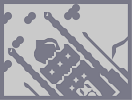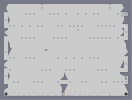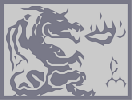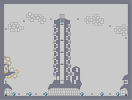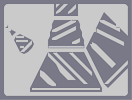Confusing Reality Escape from rising lava Honorably Dragon He's pointing at you Traffic City Crome Cubes

Pages: (0)

### Looks good so far,

Love the dragon! 4

### Beautiful

Really beautiful.
4.5/5

### ok....

tryed a lot of greens. its possible, but xxx-treme laggy xP
ill see if i make such a dragon... it eats a lot of time...

### oh..

ill try it, but im not shure if its possible...

### i think its good as it is

looks like charcoal in the background almost (to me anyways)
but seeing your other work, its probably only going to get a whole lot better
Can i suggest a classic green dragon? no one has done that before, probably because noone has found a light green

### shame

the purple didnt work :( but its still gonna look ace. i am still thinking that the part where the dragon sits on the rock is a little messy and i see im not the only one - but its hard to see from the thumb so its not really a problem. i no longer think the face is too thin :)

do something else?

### Hoppy, you should ask about that in the N Help forum.

http://metanet.2.forumer.com/index.php?s=558d5e48ce6642afb6c395cd19c8d3ea&showforum=23

### Hey

i just need some help
u probs dnt know
but
wen i play on N game
my n jumps lyk 3 secs after i clik space and same
with pressing right n left
do u know wts wrong?

5/5

Can't wait!

### cant wait to see the end result

my nails will be non existent until it comes out. 5/5

### *Checks out all maps made by *Dragon_moon"*

Wow! I've got to say that you're one impressive N artist!
Oh, there WAS a yin-yang... That's pretty cool too!

### This is how i wanted it.

In my opinion, these comments are more importand than a rating of 4.5/5 from 200 people. from this comments i can learn what i can change and where i have to keep on working.
thx

@apg just search a little in my maps, youll find a yin-yang. ;D

To the picture: i had problems with the size of the N-screen. so i had to ahm, 'comprimise' the body a little bit. and another thing: in the 1st step i wrote, that ill make the clouds purple. i tryed diffrent purples but they all dont look like that, id imagined. so ill make the clouds yellow-orange again. =D

### good starting.

I think it's a little confuse in the middle (body of the dragon)... The neck is really close to the left wing, and it's blending a little (that's what we call in french "Fu*k the flee", means "searching the very little detail that no one will ever pay attention to"), but well, hey, you wanted comments ! ;)
Very good job.
I'm waiting to see the ending... :)

### I agree with mc_george.

But by looking at the first Afternoondragon, everyone knows that the final part _will_ be bitesized and rated at least 4.5/5 by, say, 200 people. But it's a good thing to post your incomplete versions here so we can give you feedback. How about making a yin-yang somewhere? The rocky surface could be improved. At the first look, I thought the dragon was swimming in an ocean. Also, the head is a little too thin. But great job nonetheless; I can't wait to see your talent.

### hey

it is alreacdy heaps good, i reckon the sky and the rock are actually really well done.... (we know u can do the dragon part superbly)

### Looks good so far

The wings need to be fixed a little and I like the tail a lot. So you're on the right track.